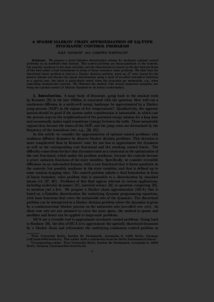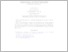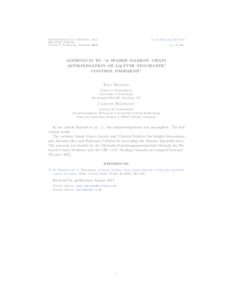Repository: Freie Universität Berlin, Math Department

# A sparse Markov chain approximation of LQ-type stochastic control problems

Banisch, Ralf and Hartmann, C. (2016) A sparse Markov chain approximation of LQ-type stochastic control problems. Math. Control Relat. F., 6 (3). pp. 363-389. ISSN 1064-8275Preview
PDF - Submitted Version
1MBPreview
PDF - Supplemental Material
134kB

## Abstract

We propose a novel Galerkin discretization scheme for stochastic optimal control problems on an indefinite time horizon. The control problems are linear-quadratic in the controls, but possibly nonlinear in the state variables, and the discretization is based on the fact that problems of this kind admit a dual formulation in terms of linear boundary value problems. We show that the discretized linear problem is dual to a Markov decision problem, prove an \$L^{2}\$ error bound for the general scheme and discuss the sparse discretization using a basis of so-called committor functions as a special case; the latter is particularly suited when the dynamics are metastable, e.g., when controlling biomolecular systems. We illustrate the method with several numerical examples, one being the optimal control of Alanine dipeptide to its helical conformation.

Item Type: Article Mathematical and Computer Sciences > Mathematics > Numerical Analysis Department of Mathematics and Computer Science > Institute of MathematicsDepartment of Mathematics and Computer Science > Institute of Mathematics > Cellular Mechanics GroupDepartment of Mathematics and Computer Science > Institute of Mathematics > BioComputing Group 1507 Carsten Hartmann 17 Feb 2015 23:30 13 Dec 2017 14:33

Repository Staff Only: item control page